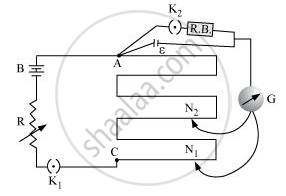Advertisement Remove all ads

# Write the Principle of Working of a Potentiometer. Describe Briefly, with the Help of a Circuit Diagram, How a Potentiometer is Used to Determine the Internal Resistance of a Given Cell. - Physics

Advertisement Remove all ads
Advertisement Remove all ads
Advertisement Remove all ads

Write the principle of working of a potentiometer. Describe briefly, with the help of a circuit diagram, how a potentiometer is used to determine the internal resistance of a given cell.

Advertisement Remove all ads

#### Solution

The working principle of a potentiometer is based on Kirchhoff’s voltage law. According to this rule, the algebraic sum of changes in voltage around any closed loop involving resistors and cells in the loop is zero.Let Φ be the potential drop per unit length in the potentiometer wire.

When only a cell is connected, the balance point is N1.

Appling Kirchhoff’s voltage law,

ε = Φ l1 [l1 = Length at which the balance point is achieved]

When some current is drawn using the resistance box, the balance point is achieved at N2.

V = Φl2

This gives,

epsi/V = l_1 / l_2

ε = I (r + R) [R = Resistance of the resistance box]

V = IR

This gives,

epsi /V = (r+R)/R

r = R (l_1/l_2  - 1)

The internal resistance of the cell can be determined by plugging-in the measured values of l1 and l2.

Concept: Potentiometer
Is there an error in this question or solution?

#### APPEARS IN

Advertisement Remove all ads
Share
Notifications

View all notifications

Forgot password?